How to Learn in 24 Hours?The Rapid Learning Movie

 Need Help? M-F: 9am-5pm(PST): Toll-Free: (877) RAPID-10 US Direct: (714) 692-2900 Int'l: 001-714-692-2900 24/7 Online Technical Support: The Rapid Support Center Secure Online Order:Need Proof? Testimonials by Our Users

 Rapid Learning Courses: MCAT in 24 Hours (2015-16) USMLE in 24 Hours (Boards) Chemistry in 24 Hours Biology in 24 Hours Physics in 24 Hours Mathematics in 24 Hours Psychology in 24 Hours SAT in 24 Hours ACT in 24 Hours AP in 24 Hours CLEP in 24 Hours DAT in 24 Hours (Dental) OAT in 24 Hours (Optometry) PCAT in 24 Hours (Pharmacy) Nursing Entrance Exams Certification in 24 Hours eBook - Survival Kits Audiobooks (MP3)

 Tell-A-Friend: Have friends taking science and math courses too? Tell them about our rapid learning system.Home »  Mathematics »  Pre-Calculus

Infinite Sequences and Series

 Topic Review on "Title": The definition of infinite sequences:  An (infinite) sequence of real numbers is a function from the positive integers n into real numbers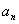,Limit of a sequence: A sequence of real numbersconverges to the number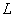if, for any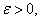there is a positive integer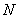such that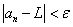for any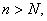is called the limit of the sequence.   Convergence of Cauchy sequences: A sequence of real numbers converges if and only if it is a Cauchy sequence.   Subsequences of a sequence: Subsequences are formed when we have a strictly increasing sequence of positive integers.   Convergence tests: The comparison test, ratio test, root test, integral test and absolute/ conditional convergence test are the tests that are used to determine the convergence of series.

Rapid Study Kit for "Title":
 Flash Movie Flash Game Flash Card Core Concept Tutorial Problem Solving Drill Review Cheat Sheet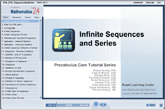"Title" Tutorial Summary : This tutorial specifically describes the concepts of infinite sequences and series. Examples are presented to with the basic operations and properties of infinite sequences. Applying some type of theorem to find the limit is important when dealing with sequences.    The properties of sequences with applications to approximation of limits are shown in the examples. The uniqueness and convergence of sequences need to be discussed before the concept of subsequences is defined. Sequences need to be bounded and monotonic so they can be classified as convergent sequences. Special sequences such as Cauchy sequences are also mentioned in this tutorial.

 Tutorial Features: Specific Tutorial Features: • Graphs showing the convergence of sequences. • Step by step analysis of the different types of convergence tests that can be used to determine is a series converges or not. Series Features: • Concept map showing inter-connections of new concepts in this tutorial and those previously introduced. • Definition slides introduce terms as they are needed. • Visual representation of concepts • Animated examples—worked out step by step • A concise summary is given at the conclusion of the tutorial.

 "Title" Topic List: Infinite sequences Arithmetic and geometric progression Definition of sequences Recursive relations Limit of a sequence Divergence of sequences Operations of a limit Bounded and monotonic sequences Definition of Cauchy sequences Convergence of Cauchy sequences Subsequences Infinite series Arithmetic sum Geometric Series Basic properties of series Comparison test Ratio, root, integral, p-series and alternating series test

See all 24 lessons in Pre-Calculus, including concept tutorials, problem drills and cheat sheets:
Teach Yourself Pre-Calculus Visually in 24 Hours